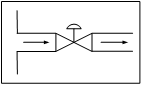# Isenthalpic Throttling & the J-T Coefficient

## ISENTHALPIC THROTTLING (FREE EXPANSION) AND THE JOULE-THOMSON COEFFICIENTThis paper is presented in four major sections.  In Section I we discuss the four basic operating regions in which isenthalpic throttling or free expansion of a pure fluid can take place.  In Sections II through IV, a fairly comprehensive study of the Joule-Thomson coefficient is conducted.  An attempt is made to develop a generalized graphical correlation for the JT inversion curve for pure fluids within the framework of the law of corresponding states.

The three-constant corresponding states equation of Miller (11) provides a very good overall or average representation of the inversion loci for light non polar gases.  In addition, an assessment is made of the accuracy of several closed-cubic equations of state for predicting the JT coefficients for nitrogen and carbon dioxide.  The four equations tested were:

1. van der Waals equation
2. Redlich-Kwong equation
3. API (modified) Soave equation
4. Peng-Robinson equation

Of the four equations tested, the Redlich-Kwong equation provided the best overall predictions of the JT coefficients for gaseous nitrogen and for both gaseous and liquid carbon dioxide.  The overall statistical deviations or trends are summarized in Tables 5, 7 and 8.

Throughout this paper, eight numerical illustrations are given which depict typical computations involving free isenthalpic expansion, the determination of the Boyle temperature and inversion temperature(s) for nitrogen from virial coefficient data, and the calculation of the inversion point and JT coefficients from a typical set of isenthalpic T vs. P data for gaseous ethylene.